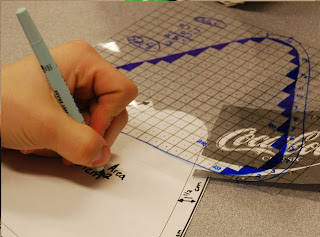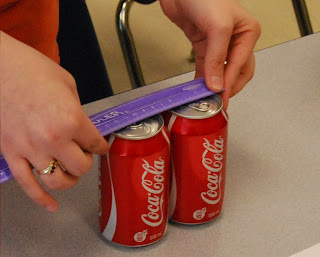Categories

# Soft Drink Project Part 4: The Math

This is the fourth in a series of posts detailing a student-posed math project. To get the full picture, please read the previous posts beginning with:

Soft Drink Project Part 1: The Framework
This post is designed to dampen the fear of math teachers. I know, because I was very afraid that the project had missed the mark until students moved into this phase. For some reason, teachers feel like they have more ability to complete a list of outcomes if they dictate the exact way, pace, and form that the learning will take. My division states they want to create life-long learners; in this model, the only lifelong learners are teachers because they must continue to do all the learning for their students day after day.

I gave a presentation on the blended project-based and problem-based system that I have adapted for my classroom this year. During the session, I claimed that great tasks involved student decisions, voice, and innovation. My goal was to show that creating an ecology where students feel comfortable expanding their problems will end up covering far more curricular topics that you would expect.
Maybe in my little world I thought this is what teachers needed to hear. I know I needed to be convinced until I saw it first hand.
The pop box designs were all unique. I honestly didn’t see that coming even though the project began with such a high volume of student influence. I thought that a “best way” would be democratically deciphered, and several groups would then move on to design it to exact specifications.
Not a chance.
On the whole, groups encountered the same mathematics. There were deviations from the status quo, but they occurred in extreme circumstances. The lesson for me (and all teachers interested) is that we can still tailor a student-driven class around a content-driven curricula.
Below are some of the mathematical skills my students encountered:
• Surface Area
• I would hope so. The initial lesson was designed to have students explore how changing the dimensions of a prism would affect the surface area. This was the starting point of the learning. Students designed several boxes and calculated areas to compare. Students were discussing the formulae for areas of triangles and rectangles–using arguments to justify their design. Triangles, circles, and various polygons were calculated. Even an irregular shape was estimated.A student estimates the SA of his newly minted shapeStudent uses grid shading to create an accurate estimate
• Measurement
• The dimensions of every shape had to be labeled. This was particularly interesting for triangles. The students had to decide which dimensions were necessary/important to include to calculate the surface area. The most eluding measurement was the diameter of the can. The bevel along the top and bottom made this an interesting problem. Students recalled their skills from a previous lesson to take the measurement.Student measures the diameter of two cans
• Pythagorean Theorem
• I wasn’t expecting to fit this into the project, but it usually manages to squeeze itself into places where triangles are present. Students often forgot that the height of any triangle is needed to calculate its area. (Well at least until they become familiar with the idea of semi perimeter). I would ask them if they had a way to calculate the height and most remembered the theorem. Few knew how to use it. I think students remember the theorem because it represents a particularly traumatic experience in school mathematics. Regardless, students found their height and calculated areas.
• Error Estimation
• Many students realized the effect that human error has on mathematics. Their centimetre rulers only contained one digit of accuracy while their tracing and cutting skills contained far less. One group found it particularly distressing that their Pythagorean Theorem calculation differed from their measurement of the height.
• Student 1 – “Does that mean the theorem is wrong?”
• Student 2 – “No, it means that our measurements are wrong.”
• Students blind recognition of mathematics is troubling. Nowhere along the line did they ever question the fact that side-squared-plus-side-squared-equals-hypotenuse-squared.
• Combinatorics
• This was pretty much exclusive to the group that created the Tetris box design. As they were drawing up possible arrangements, I asked them how many possible arrangements existed. They could then make an objective choice as to which to bring to life. Essentially this was a tiling problem with irregular tiles composed of 4 squares.
• Algebra
• The student who designed the triangular prism cans to fit snugly inside the triangular prism box was well on his way to completion when I asked him how big each of his cans were. After a discussion, and a quick Google-ing to see the conversion between cubed centimetres and millilitres, we decided that we should shift the height of the cans so they held exactly 355mL. The area of the base of his can was fixed at 21.35 squared centimetres. (An equilateral triangle with sides of 7cm). He knew he wanted the volume to be 355 cubic centimetres. He recalled our prior tasks with prisms to set up the following equation:
• Volume = Area of base * height = 355
• Volume = 21.35 * h = 355
• He then went on to experience his very first real application of algebra. He made sure to mention to everyone who would listen that his cans contained exactly the same amount of liquid as the regular cans.The last 355mL triangular prism can is created (with help from my fabulous EA)
Other, specialized, areas of mathematics emerged as well. One group used their knowledge of computer assisted drafting to complete their design; another group discovered that a number can be considered “triangular” just like some numbers are considered “square”. I was initially worried that the mathematics would take a back seat to colouring, cutting, and taping, but this could not have been further from the truth.
Throughout the entire process, the mathematics remained front-and-center. One colleague asked if I considered the task to be student-centered. I think that the project’s room for student innovation created a task that was mathematics centered. Isn’t that the way a classroom should operate?
Continue Reading –> Soft Drink Project Part 5: The Show
NatBanting